## Friday, 3 October 2014

### KIRCHOFF'S RULE PROBLEM EXPLANATION TO DETERMINE UNKNOWN CURRENTS

Consider the circuit below: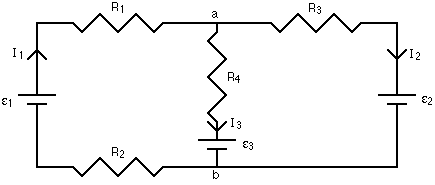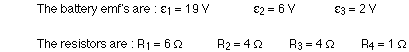Step 1 of the branch current method has already been done. The currents have been labeled in each branch of the circuit, and the directions are shown with arrows. Again, you don't have to be sure of these directions at this point. Simply choose directions, and if any of the currents come out to have negative signs, all it means is that the direction of that current is opposite to the way you've shown on your diagram.
Applying step 2 of the branch current method means looking at the junctions, and writing down a current equation. At junction a, the total current coming in to the junction equals the total current flowing away. This gives:
at junction a : I1 = I2 + I3
If we applied the junction rule at junction b, we'd get the same equation. So, applying the junction rule at one of the junctions is all we need to do. In some cases you will need to get equations from more than one junction, but you'll never need to get an equation for every junction.
There are three unknowns, the three currents, so we need to have three equations. One came from the junction rule; the other two come from going to step 3 and applying the loop rule. There are three loops to use in this circuit: the inside loop on the left, the inside loop on the right, and the loop that goes all the way around the outside. We just need to write down loop equations until each branch has been used at least once, though, so using any two of the three loops in this case is sufficient.
When applying the loop equation, the first step is to choose a starting point on one loop. Then walk around the loop, in either direction, and write down the change in potential when you go through a battery or resistor. When the potential increases, the change is positive; when the potential decreases, the change is negative. When you get back to your starting point, add up all the potential changes and set this sum equal to zero, because the net change should be zero when you get back to where you started.
When you pass through a battery from minus to plus, that's a positive change in potential, equal to the emf of the battery. If you go through from plus to minus, the change in potential is equal to minus the emf of the battery.
Current flows from high to low potential through a resistor. If you pass through a resistor in the same direction as the current, the potential, given by IR, will decrease, so it will have a minus sign. If you go through a resistor opposite to the direction of the current, you're going from lower to higher potential, and the IR change in potential has a plus sign.
Keeping all this in mind, let's write down the loop equation for the inside loop on the left side. Picking a starting point as the bottom left corner, and moving clockwise around the loop gives: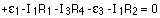Make sure you match the current to the resistor; there is one current for each branch, and a loop has at least two branches in it.
The inner loop on the right side can be used to get the second loop equation. Starting in the bottom right corner and going counter-clockwise gives: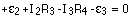Plugging in the values for the resistances and battery emf's gives, for the three equations: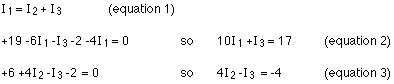The simplest way to solve this is to look at which variable shows up in both loop equations (equations 2 and 3), solve for that variable in equation 1, and substitute it in in equations 2 and 3.
Rearranging equation 1 gives:Substituting this into equation 2 gives: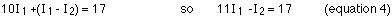Making the same substitution into equation 3 gives: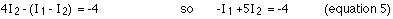This set of two equations in two unknowns can be reduced to one equation in one unknown by multiplying equation 4 by 5 (the number 5, not equation 5!) and adding the result to equation 5.Substituting this into equation 5 gives:
I2 = ( -4 + 1.5 ) / 5 = -0.5 A
The negative sign means that the current is 0.5 A in the direction opposite to that shown on the diagram. Solving for the current in the middle branch from equation 1 gives:
I3 = 1.5 - (-0.5) = 2.0 A
An excellent way to check your answer is to go back and label the voltage at each point in the circuit. If everything is consistent, your answer is fine. To label the voltage, the simplest thing to do is choose one point to be zero volts. It's just the difference in potential between points that matters, so you can define one point to be whatever potential you think is convenient, and use that as your reference point. My habit is to set the negative side of one of the batteries to zero volts, and measure everything else with respect to that.In this example circuit, when the potential at all the points is labeled, everything is consistent. What this means is that when you go from junction b to junction a by any route, and figure out what the potential at a is, you get the same answer for each route. If you got different answers, that would be a big hint that you did something wrong in solving for the currents. Note also that you have to account for any of the currents coming out to be negative, and going the opposite way from what you had originally drawn.
One final note: you can use this method of circuit analysis to solve for more things than just the current. If one or more of the currents was known (maybe the circuit has an ammeter or two, measuring the current magnitude and direction in one or two branches) then an unknown battery emf or an unknown resistance could be found instead.